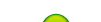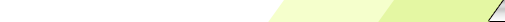Friday, 2020-02-28BioInfo Pakistan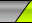Home | Sign Up | Log In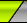Welcome Guest | RSS
Section categories
 Related Subjects This category includes brief overview of all related subjects. Defining BioInformatics In this section we tried to briefly explain what bioinformatics is ? Unviersities This contains information about universities that are offering bioinformatics degree programs. Resources Contains information about bioinformatics resources including databases, tools and techniques. Algorithms This category includes some of the basic algorithms that are usually used by bioinformaticians.
 Our poll Pakistani Student Yes No Total of answers: 0
 Chat Box Only authorized users can post messages
 StatisticsTotal online: 1 Guests: 1 Users: 0
Home » 2011 » August » 20 » Baum–Welch Algorithm
10:14 PM
Baum–Welch Algorithm

 Baum–Welch Algorithm1. Description:   In electrical engineering, computer science, statistical computing and bioinformatics, the Baum–Welch algorithm is used to find the unknown parameters of a hidden Markov model (HMM). It makes use of the forward-backward algorithm and is named for Leonard E. Baum and Lloyd R. Welch.2. Explanation:   The Baum–Welch algorithm is a particular case of a generalized expectation- maximization (GEM) algorithm. It can compute maximum likelihood estimates and posterior mode estimates for the parameters (transition and emission probabilities) of an HMM, when given only emissions as training data.   For a given cell Si in the transition matrix, all paths to that cell are summed. There is a link (transition from that cell to a cell Sj). The joint probability of Si, the link, and Sj can be calculated and normalized by the probability of the entire string. Call this χ.   Now, calculate the probability of all paths with all links emanating from Si. Normalize this by the probability of the entire string. Call this σ.   Now divide χ by σ. This is dividing the expected transition from Si to Sj by the expected transitions from Si. As the corpus grows, and particular transitions are reinforced, they will increase in value, reaching a local maximum. No way to ascertain a global maximum is known.3. General Idea: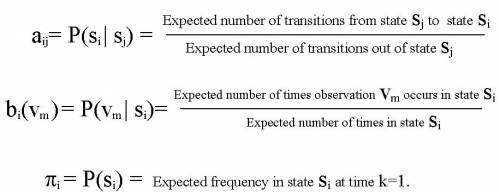4. Expectation Step:   Define variable xk(i,j) as  the probability of being in state si at time k and in state sj at  time k+1, given the observation sequence o1 o2 ... oK. xk(i,j)= P(qk= si  , qk+1= sj  | o1 o2 ... oK)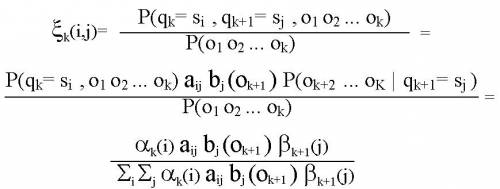Define variable gk(i) as  the probability of being in state si at time k, given the observation sequence o1 o2 ... oK.                 gk(i)= P(qk= si | o1 o2 ... oK)   1. We calculated P(qk= si  , qk+1= sj  | o1 o2 ... oK) and gk(i)= P(qk= si | o1 o2 ... oK).  2. Expected number of transitions from state si to state sj = Sk xk(i,j)   3. Expected number of transitions out of state si = Sk  gk(i)   4. Expected number of times observation vm occurs in state si = Sk gk(i) , k is such that ok= vm    5. Expected frequency in state si at time k=1 : g1(i). 5. Maximization Step:
Category: Algorithms | Views: 1255 | Added by: Ansari | Rating: 0.0/0